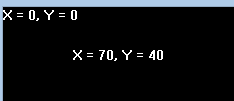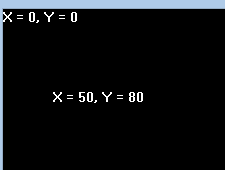# moveto() function in C

The header file graphics.h contains moveto() function which changes the current position to (x, y)

Syntax :

``` void moveto(int x, int y);
```

Examples :

```Input : x = 70, y = 40
Output :Input : x = 50, y = 80
Output :```

Below is the implementation of moveto() function:

 `// C Implementation for moveto() ` `#include ` `#include ` ` `  `// driver code ` `int` `main() ` `{ ` `    ``// gm is Graphics mode which is ` `    ``// a computer display mode that ` `    ``// generates image using pixels. ` `    ``// DETECT is a macro defined in ` `    ``// "graphics.h" header file ` `    ``int` `gd = DETECT, gm; ` `    ``char` `arr; ` ` `  `    ``// initgraph initializes the ` `    ``// graphics system by loading a ` `    ``// graphics driver from disk ` `    ``initgraph(&gd, &gm, ``""``); ` ` `  `    ``// sprintf stands for “String print”. ` `    ``// Instead of printing on console, it ` `    ``// store output on char buffer which ` `    ``// are specified in sprintf ` `    ``sprintf``(arr, ``"X = %d, Y = %d"``, getx(), ` `                                 ``gety()); ` ` `  `    ``// outtext function displays text at ` `    ``// current position. ` `    ``outtext(arr); ` ` `  `    ``// moveto function ` `    ``moveto(70, 40); ` ` `  `    ``// The function getx returns the X ` `    ``// coordinate of the current position. ` `    ``// The function gety returns the y ` `    ``// coordinate of current position. ` `    ``sprintf``(arr, ``"X = %d, Y = %d"``, getx(),  ` `                                 ``gety()); ` ` `  `    ``outtext(arr); ` ` `  `    ``getch(); ` ` `  `    ``// closegraph function closes the ` `    ``// graphics mode and deallocates ` `    ``// all memory allocated by ` `    ``// graphics system . ` `    ``closegraph(); ` ` `  `    ``return` `0; ` `} `

Output :

``````

My Personal Notes arrow_drop_upIn love with a semicolon because sometimes i miss it so badly)

If you like GeeksforGeeks and would like to contribute, you can also write an article using contribute.geeksforgeeks.org or mail your article to contribute@geeksforgeeks.org. See your article appearing on the GeeksforGeeks main page and help other Geeks.

Please Improve this article if you find anything incorrect by clicking on the "Improve Article" button below.

Article Tags :
Practice Tags :

Be the First to upvote.

Please write to us at contribute@geeksforgeeks.org to report any issue with the above content.